# Uniqueness Test

The Uniqueness Tests are a set of techniques that avoid the various deadly patterns, assuming that the given Sudoku is valid. The patterns involved are often easy to recognize, but it should be noted that since a deadly pattern will never occur in a valid Sudoku, these techniques are not strictly necessary to avoid them -- the puzzle can always be solved by other means.

This article focuses on the uniqueness tests used to avoid the Unique Rectangle deadly pattern. The techniques used to avoid the Bivalue Universal Grave (BUG) and BUG Lite patterns are discussed in the BUG and BUG Lite articles.

Generally, the Unique Rectangle tests consider four cells that form a rectangle whose common candidates are two digits, with one or more of these cells containing extra candidates as well. The 4 cells in the corners of the rectangle belong to exactly 2 rows, 2 columns and 2 boxes. To avoid this deadly pattern, at least one of the extra candidates must be placed.

### Uniqueness Tests

Uniqueness Test 1

Uniqueness Test 1 is the simplest among all uniqueness tests. It can be stated as follows:

If there is only one cell in the rectangle that contains extra candidates, then the common candidates can be eliminated from that cell.

An example below: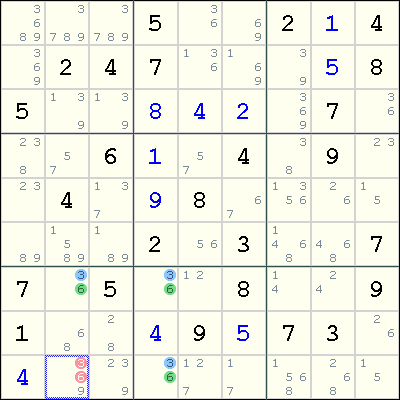Suppose r9c2 contains only either 3 or 6, i.e. having 3 and 6 as its only candidates. Then the four cells r7c2, r7c4, r9c4 and r9c2 forms a Unique Rectangle deadly pattern on the digits 3 and 6, which makes the puzzle invalid. Therefore, both 3 and 6 can be eliminated from r9c2.

Uniqueness Test 2

This test can be stated as follows:

Suppose exactly two cells in the rectangle have exactly one extra candidate X, and both cells lie on one side of the rectangle. Then X can be eliminated from the cells seen by both of these cells. Note the similarity with Uniqueness Test 5 described later.

Uniqueness Test 2 can be illustrated in the example below: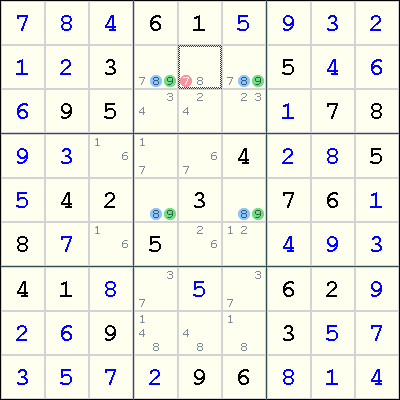To avoid the Unique Rectangle for the digits 8 and 9, either r2c4 or r2c6 must contain the digit 7. Therefore, 7 can be eliminated from any cell that is seen by both r2c4 and r2c6.

Uniqueness Test 3

This test can be stated as follows:

Suppose exactly two cells in the rectangle, both located on the one side of the rectangle, have extra candidates. By treating these two cells as one node, find k - 1 other cells (as nodes) in the same house as these two cells so that the union of the candidates for these k cells has exactly k unique digits. Then the Naked Subset rule can be applied eliminate these k digits from the other cells in the house.

In a way, Uniqueness Test 3 can be seen as an extension of Uniqueness Test 2. When k = 1, Test 3 reduces to Test 2.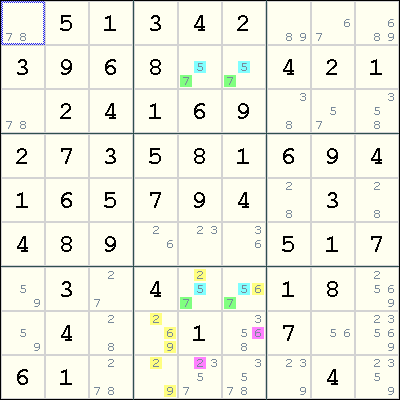By treating r7c56, r8c4 and r9c4 as a Naked Triple on the digits 2, 6, and 9, we can eliminate 6 from r8c6 and 2 from r9c5.

Uniqueness Test 4

This test can be stated as follows:

Suppose exactly two cells in the rectangle contain extra candidates, and they are located on the same side of the rectangle. Suppose the common candidates are U and V, and none of the cells seen by both of these cells contain U. Then V can be eliminated from these two cells.

An example below:In this example, observe that the common candidates are 2 and 7, and the extra candidates lie in r9c5 and r9c8. However, in row 9, the digit 7 must be placed in either r9c5 or r9c8. Since exactly two digits must be placed in r9c5 and r9c8, one being the digit 7 and the other coming from one of the extra candidates, there is no room for these two cells to contain the digit 2. Thus, 2 can be eliminated from both r9c5 and r9c8.

Uniqueness Test 5

Uniqueness Test 5 is very similar to Uniqueness Test 2, and can be stated as follows:

Suppose exactly two cells in the rectangle have exactly one extra candidate X, and both cells are located diagonally across each other in the rectangle. Then X can be eliminated from the cells seen by both of these cells.

However, it appears that no example has been found for this test.

Some players will also call the following variant as Uniqueness Test 5:

Suppose exactly three cells in the rectangle have exactly one extra candidate X. Then X can be eliminated from the cells seen by all three cells.Note the unique rectangle where rows 2 and 6, and columns 8 and 9 intersect. The common candidates are 5 and 9, and three of these cells, r2c8, r2c9 and r6c8, have an extra candidate 6. To avoid the deadly pattern, at least one of r2c8, r2c9 and r6c8 must contain a 6. As r1c8 sees all of these three cells, we can eliminate 6 from r1c8.

Uniqueness Test 6

Uniqueness Test 6 is very similar to Uniqueness Test 4, and can be stated as follows:

Suppose exactly two cells in the rectangle contain extra candidates, and they are located diagonally across each other in the rectangle. Suppose the common candidates are U and V, and none of the other cells in the two rows and two columns containing the rectangle contain U. Then V can be eliminated from these two cells.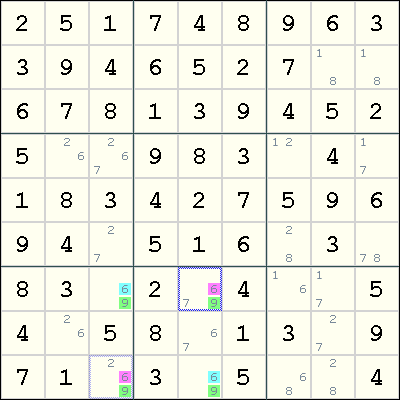An unique rectangle on the digits 6 and 9 plus two other candidates can be found in the intersections of rows 7 and 9, and the columns 3 and 5. In these rows and columns, the candidates for digit 9 can only be found in r7c3, r7c5, r9c5 and r9c3. So in r7c5 and r9c3, one of these cells must be a 9 and the other coming from one of the extra candidates. So the digit 6 can be safely eliminated from these two cells.

### Unique Rectangle and Implications

Unique Rectangle and Forcing Chains

A Unique Rectangle with extra candidates means that at least one of the extra candidates must be placed in its cell. This means that each of the extra candidates can be the starting point of a Forcing Chain.In this example, since either r9c1 contains 3 or r8c9 contains 2, we can form two Forcing Chains:

r9c1=3 => r4c1<>3 => r4c1=5 => r6c2<>5 => r6c2=7 => r5c2<>7
r8c9=2 => r5c9<>2 => r5c9=7 => r5c2<>7

Both chains result in r5c2<>7, so we can eliminate 7 from r5c2.

Unique Rectangle as Strong Link

When a Unique Rectangle has exactly two extra candidates, then the elimination of any one candidate means that the other candidate must be placed. This implies that these two candidates have a strong link. Note that this strong link does not necessarily encode a weak link, but the strong-only link may be sufficient to make an inference that causes eliminations in other cells.This is the same example as above, but recast to use a loop to make the same elimination. First, observe that we have a strong link between 3 at r9c1 and 2 at r8c9. This enables us to construct the following Alternating Inference Chain (in Eureka notation):

(7)r5c2-(7=5)r6c2-(5=3)r4c1-(3)r9c1=(2)r8c9-(2=7)r5c9-(7)r5c2 => r5c2<>7

This also results in 7 being eliminated from r5c2.

﻿

## Sudoku strategies

﻿## Printable Sudoku## Sudoku Links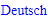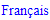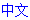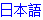﻿